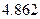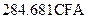Chapter 19, Problem 9SCQ### Principles of Economics 2e

2nd Edition
Steven A. Greenlaw; David Shapiro
ISBN: 9781947172364

#### Solutions

Chapter
Section### Principles of Economics 2e

2nd Edition
Steven A. Greenlaw; David Shapiro
ISBN: 9781947172364
Textbook Problem

# The Central African Republic has a GDP of 1,107,689 million CFA francs and a population ofmillion. The exchange rate isfrancs per dollar. Calculate the GDP per capital of Central African Republic.

To determine

The Gross Domestic Product (GDP) per capita of Central African Republic.

Explanation

GDP per capita of a country is defined as GDP divided by population size of that country.

The formula for calculating GDP per capita is:

GDPpercapita=GDPPopulationSize

Given Information:

GDP of Central African Republic = 1,107,689 million CFA francs

Population of Central African Republic = 4.862 million

Exchange rate of CFA francs to dollar = 284.681 CFA francs/\$

Using the formula above, we calculate the GDP per capita of Central African Republic.

Calculation:

GDPpercapita=1,107,6894.862=227,825

### Still sussing out bartleby?

Check out a sample textbook solution.

See a sample solution

#### The Solution to Your Study Problems

Bartleby provides explanations to thousands of textbook problems written by our experts, many with advanced degrees!

Get Started